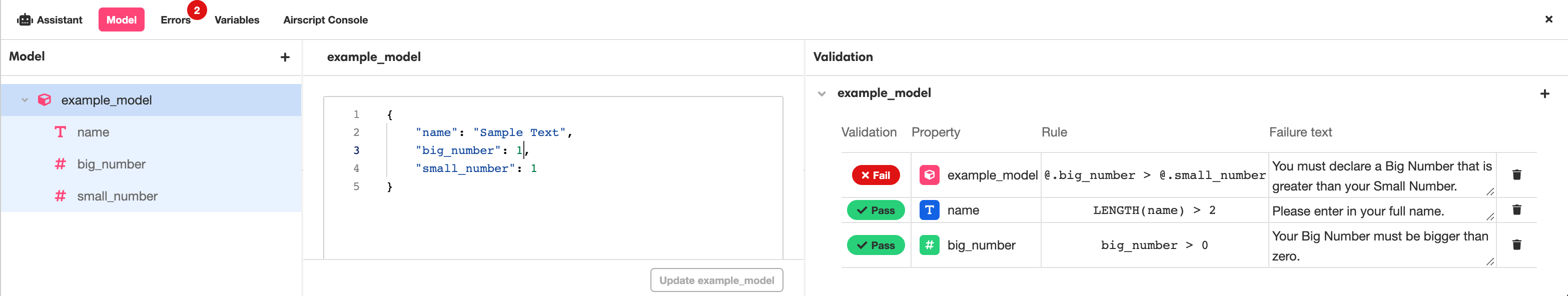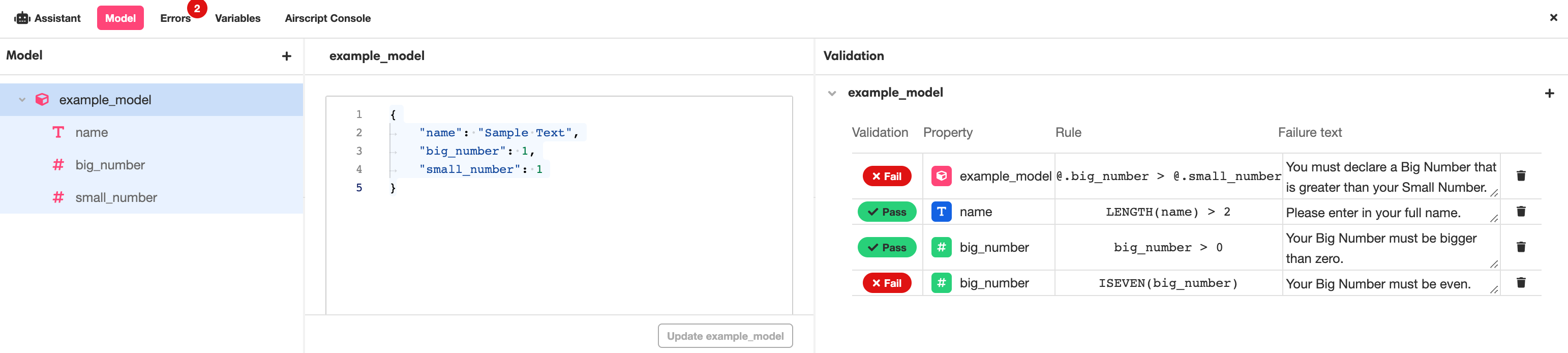The function RUN_VALIDATIONS runs the Validations attached to a Data Model.

This function references a configured Data Model to test a given Object against the Data Model's validation requirements. It returns an Object that structures the Error Text associated with any of the relevant Validations that the given Object violates.

📘

Using the RUN_VALIDATION requires at least configured Data Model.

For more on what Data Models are and how to configure them, see Data Model.

### Declaration

``````RUN_VALIDATIONS(model_object, model_name, property_names) -> validation_results
``````

### Parameters

model_object (required, type: Object)

An Object. The structure of this Object should correspond with the structure of the given Data Model.

model_name (required, type: string)

The name of a Model configured in the Data Model Editor.

property_names (optional, type: List of strings)

A List of the given Data Model's properties. Should a value be given for property_names, the Validations assigned to the Listed properties will be the only validation tests run.

If no value is given for property_names, all Validations associated with the given Data Model will be run, including those that span the Model as a whole.

### Return Values

validation_results (type: Object)

An Object that structures the Error Text associated with any of the relevant Validations that the given Object violates.

This Object consists of properties corresponding to any properties that failed at least one of their associated Validations, and each of those properties contains a List of relevant Error Texts (type: string). If the given Object violates no Validations, then the returned Object will be empty.

### Examples

Assume all of our examples have access to the following Data Model, example_model. Note that example_model has been configured with three Validation Rules: one that applies to the Model as a whole, one that applies only to the `"name"` property, and one that applies only to the `"big_number"` property:Assume also that all of our examples have access to the following Object, `example_object`. Note that `example_object` violates all three of the Validation Rules defined by example_model:

``````example_object -> {
"name": "CJ",
"big_number": 0,
"small_number": 2
}

``````

The following example tests `example_object` against all of example_model's Validations and returns an Object that structures the Error Text associated with all three of the violated Validations. Note how the strings of Error Text are sorted into Lists that correspond to the name of the Model or property that they are associated with:

``````RUN_VALIDATIONS(example_object, "example_model") -> {
"\$\$example_model": [
"You must declare a Big Number that is greater than your Small Number."
],
"name": [
],
"big_number": [
"Your Big Number must be bigger than zero."
]
}

``````

It is also possible to test Objects against only the Validations that are associated with certain Model properties. For instance, the following example only tests `example_object` against the Validations example_model has tied to the the `"name"` property:

``````RUN_VALIDATIONS(example_object, "example_model", [ "name" ]) -> {
"name": [
]
}

``````

When Objects are only tested against property-level Validations, Model-level Validation Rules are not applied, even if they would be applicable. For instance, the following example only tests `example_object` against the Validations tied to the `"big_number"` and `"small_number"` properties. Note how the Failure Text associated with the Model-level Validation does not appear, even though that rule defines how `"big_number"` and `"small_number"` are allowed to compare to each other:

``````RUN_VALIDATIONS(example_object, "example_model", [ "big_number", "small_number" ]) -> {
"big_number": [
"Your Big Number must be bigger than zero."
]
}

``````

The Objects given to the RUN_VALIDATIONS function do not need to contain every property associated with the given Data Model, just the relevant properties. For instance, the following example tests the Object `{"name": "CJ"}` against the Validations example_model has tied to the the `"name"` property:

``````RUN_VALIDATIONS({"name": "CJ"}, "example_model", [ "name" ]) -> {
"name": [
]
}
``````

If the the RUN_VALIDATIONS function is given an Object that violates none of the relevant Validations, then the returned Object will be empty. For instance, the following example only tests the Object `{"name": "Jane Doe"}` against the Validations example_model has tied to the the "name" property, and "Jane Doe" is a valid value for the `"name"` property:

``````RUN_VALIDATIONS({ "name": "Jane Doe" }, "example_model", [ "name" ]) -> {}
``````

Up until this point, we've been dealing with a Data Model that had no more than one Validation associated with each property, but that need not be the case. If we reconfigure example_model so that it has two Validations associated with the `"big_number"` property, like so:then in cases where both Validations are violated, both of their associated Failure Texts will be returned in the same List:

``````RUN_VALIDATIONS({ "big_number": -1 }, "example_model", [ "big_number" ]) -> {
"big_number": [
"Your Big Number must be bigger than zero.",
"Your Big Number must be even."
]
}

``````

# Discussion

The RUN_VALIDATIONS function is most commonly used when using the Form Web Control to automatically generate forms out of Data Models. Using the RUN_VALIDATIONS function to manage validation and error messages greatly expedites the process of building a form, especially if the form in question has many or complicated validation requirements. For more on how this works, see Making Forms from Data Models.